Ex 11.2

Chapter 11 Class 11 - Intro to Three Dimensional Geometry
Serial order wise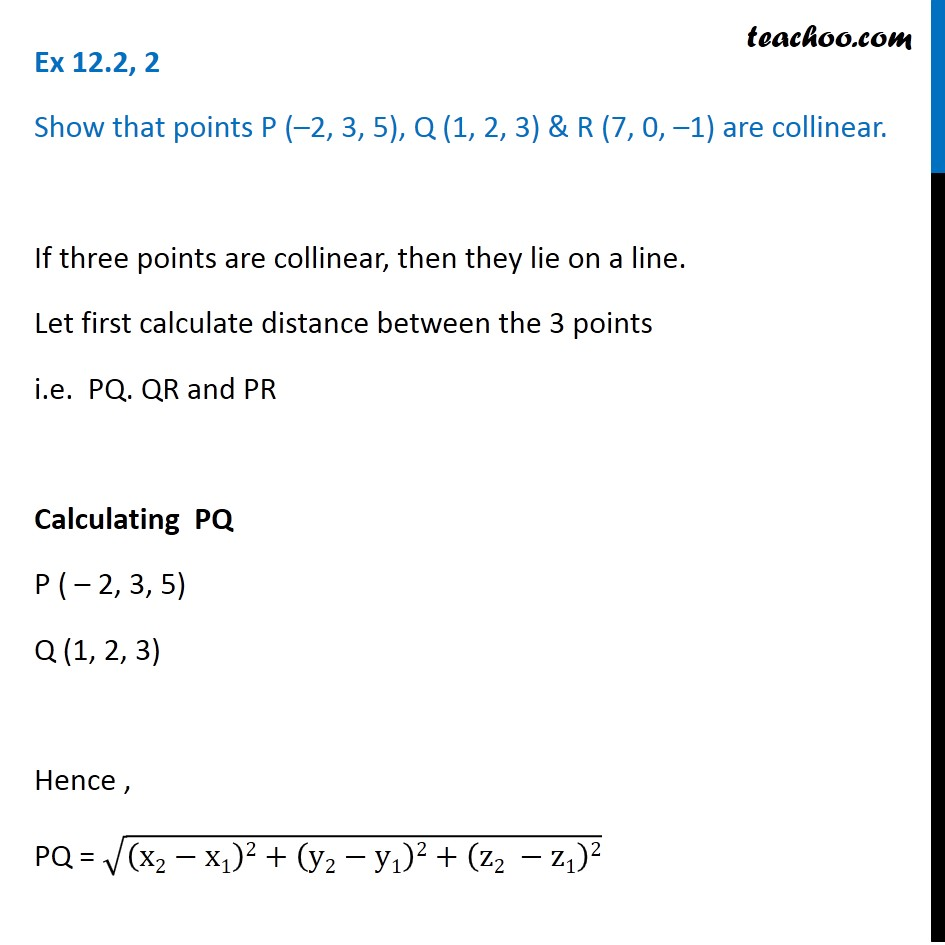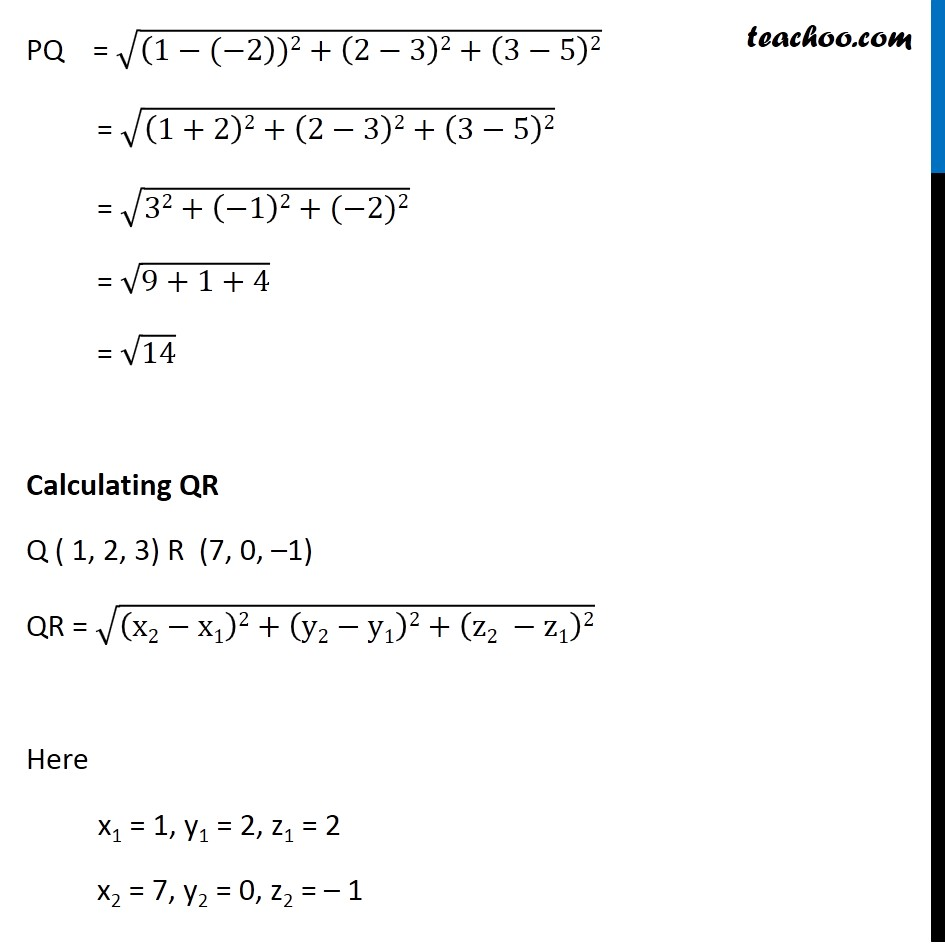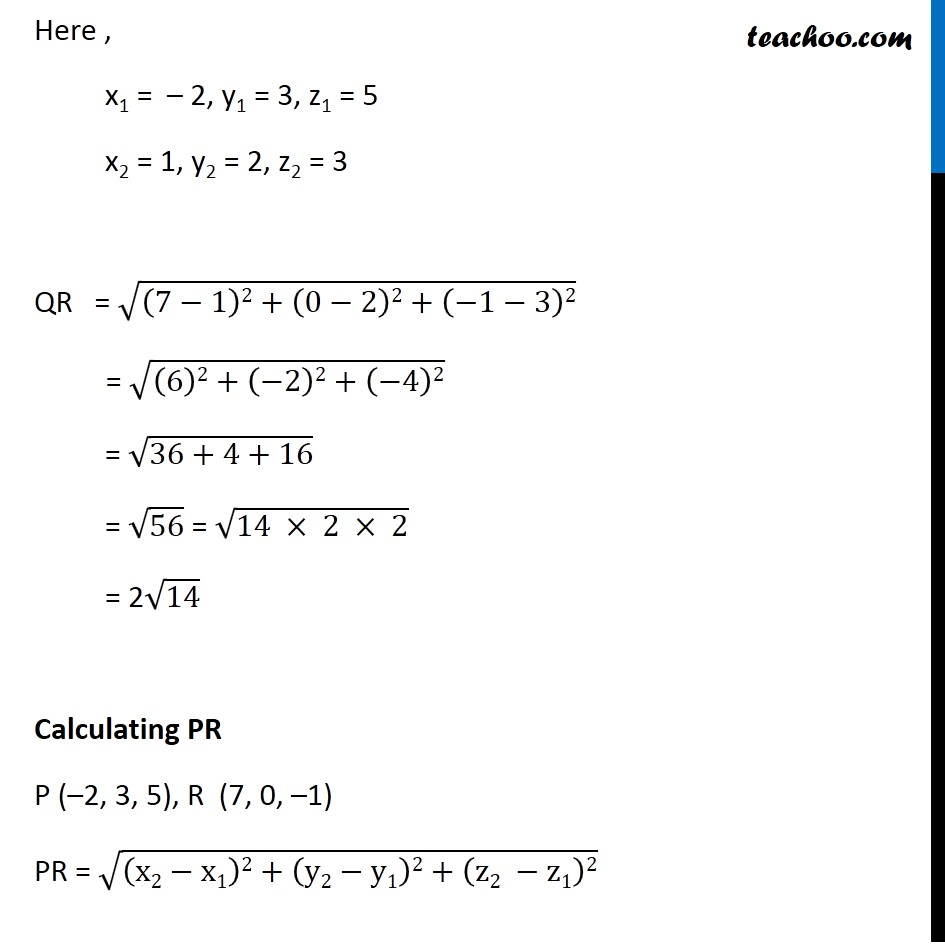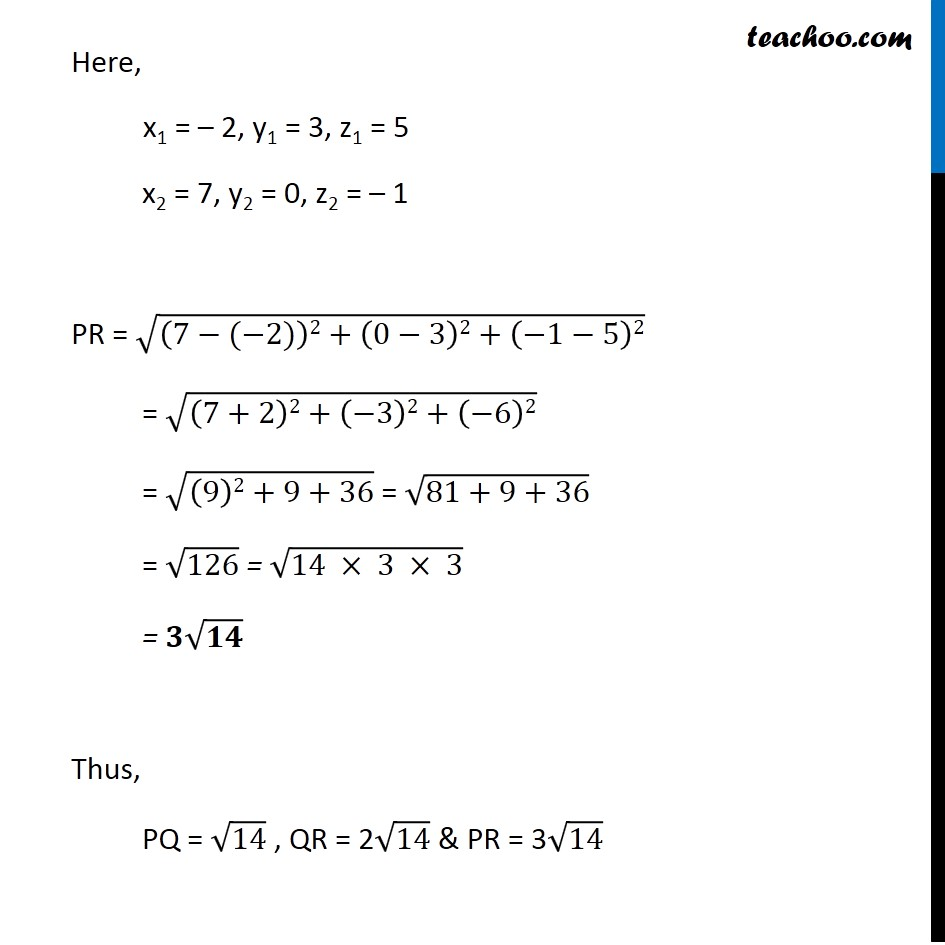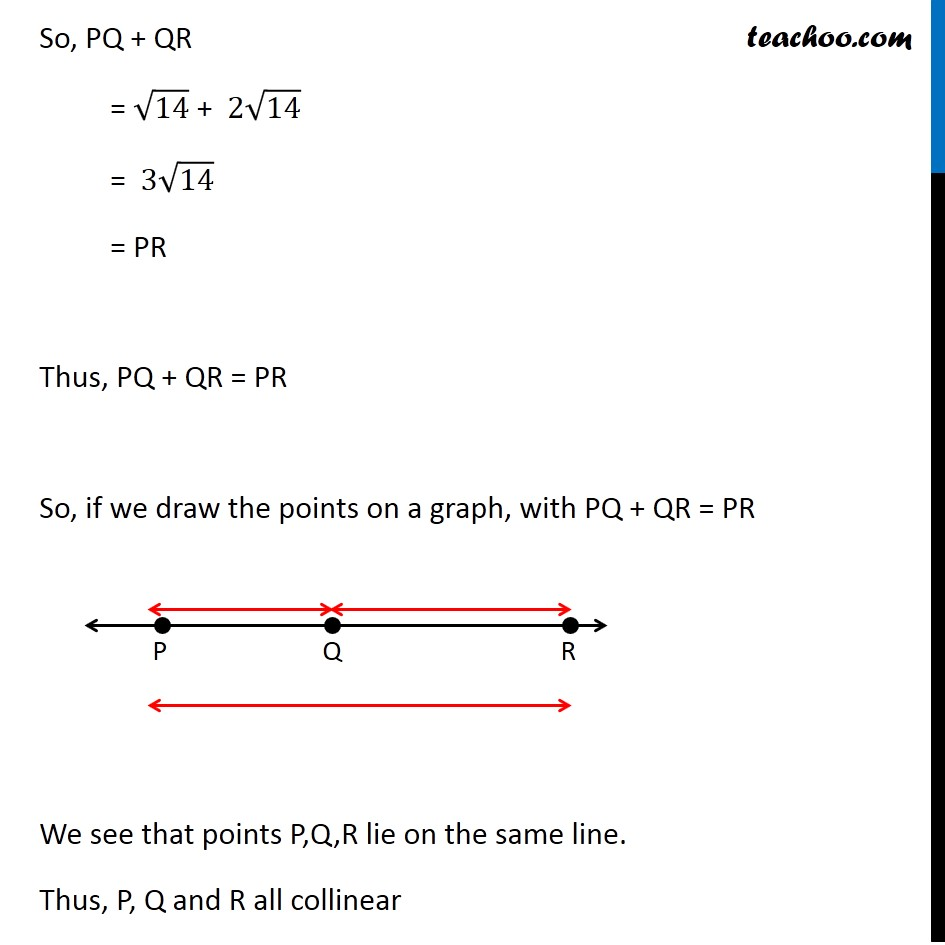Learn in your speed, with individual attention - Teachoo Maths 1-on-1 Class

### Transcript

Ex 11.2, 2 Show that points P (–2, 3, 5), Q (1, 2, 3) & R (7, 0, –1) are collinear. If three points are collinear, then they lie on a line. Let first calculate distance between the 3 points i.e. PQ. QR and PR Calculating PQ P ( – 2, 3, 5) Q (1, 2, 3) Hence , PQ = √((x2−x1)2+(y2−y1)2+(z2 −z1)2) PQ = √((1−(−2))2+(2−3)2+(3−5)2) = √((1+2)2+(2−3)2+(3−5)2) = √(32+(−1)2+(−2)2) = √(9+1+4) = √14 Calculating QR Q ( 1, 2, 3) R (7, 0, –1) QR = √((x2−x1)2+(y2−y1)2+(z2 −z1)2) Here x1 = 1, y1 = 2, z1 = 2 x2 = 7, y2 = 0, z2 = – 1 Here , x1 = – 2, y1 = 3, z1 = 5 x2 = 1, y2 = 2, z2 = 3 QR = √((7−1)2+(0−2)2+(−1−3)2) = √((6)2+(−2)2+(−4)2) = √(36+4+16) = √56 = √(14 × 2 × 2) = 2√14 Calculating PR P (–2, 3, 5), R (7, 0, –1) PR = √((x2−x1)2+(y2−y1)2+(z2 −z1)2) Here, x1 = – 2, y1 = 3, z1 = 5 x2 = 7, y2 = 0, z2 = – 1 PR = √((7−(−2))2+(0−3)2+(−1−5)2) = √((7+2)2+(−3)2+(−6)2) = √((9)2+9+36) = √(81+9+36) = √126 = √(14 × 3 × 3) = 𝟑√𝟏𝟒 Thus, PQ = √14 , QR = 2√14 & PR = 3√14 So, PQ + QR = √14 + 2√14 = 3√14 = PR Thus, PQ + QR = PR So, if we draw the points on a graph, with PQ + QR = PR We see that points P,Q,R lie on the same line. Thus, P, Q and R all collinear